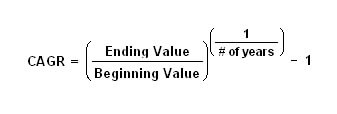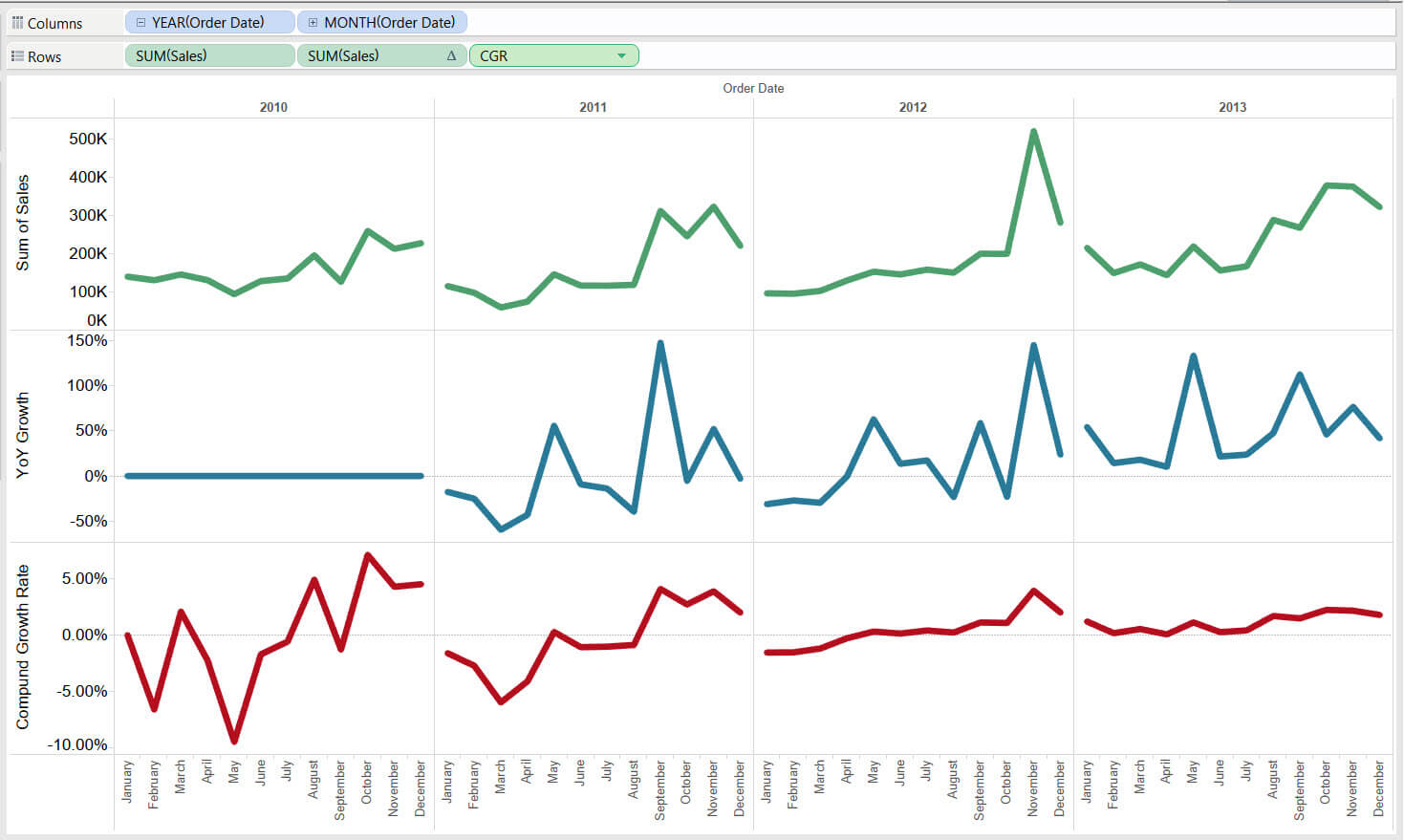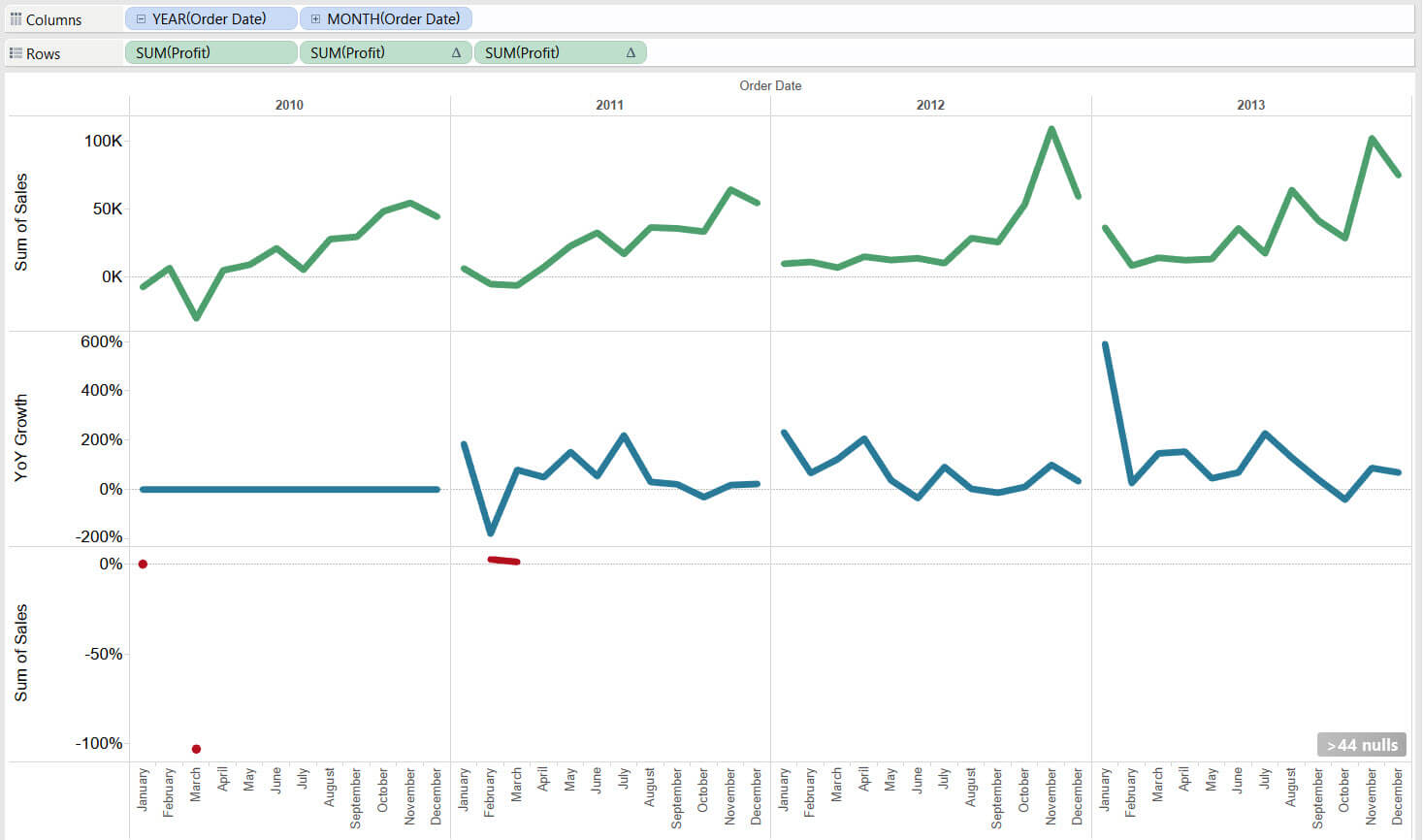## Compound Growth Rate quick table calculation in Tableau

by Naledi Hollbruegge

One of the options amongst your quick table calculations in to compute the ‘compound growth rate’ (CGR). The CGR is a measure of growth over multiple time periods. It denotes the growth rate from the initial value to the final value, assuming that the investment has compounding growth over the time period.

A compunding growth rate is calculated with the following formula.This translates to Tableau as:

POWER(ZN(SUM([Sales]))/LOOKUP(ZN(SUM([Sales])),-[N Years]), ZN(1/[N Years])) – 1

or

((End Value/Beginning Value)^(1/n)) – 1

Luckily, we don’t have to create a calculated field manually every time we want to figure out the CGR. We just right click our measure pill in the view > select ‘Quick table calculation’ > ‘Compoung growth Rate’. Note that this option will only be available once you have at least one date field in the view.

In the example below the sum of sales, year over year growth (relative to 2010) and CGR (compute using ‘Order Date’) have been plotted. You can see how the CGR becomes less jagged over time and comes closer to predicting a steady growth rate, as it takes into account the values of each month across the years.Note that measures that contain both positive and negative values across the chosen time period are problematic in this context. This is because the calculation is taking the root of a number and if you have a negative start value and a positive end value then this would mean taking the root of a negative number. (http://www.experiglot.com/2008/09/15/calculating-cagr-compound-annual-growth-rate-when-the-beginning-value-is-negative/). When using profit for instance, the first sum of sales value is negative and subsequently only those values that are also negative are plotted. Those calculations involving positive profit result in null values.Looking at your CGR may be useful when you have highly fluctuating values or data that spans a long period of time, as it allows you to tease out a general trend.

Sources:Naledi Hollbruegge

Thu 25 Aug 2016

Tue 23 Aug 2016

Mon 15 Aug 2016